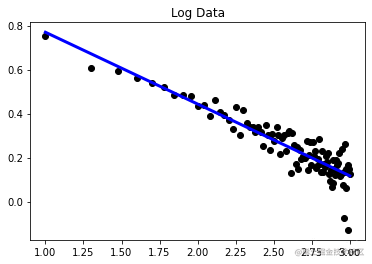# Python数据可视化系列之幂律分布

1.幂律分布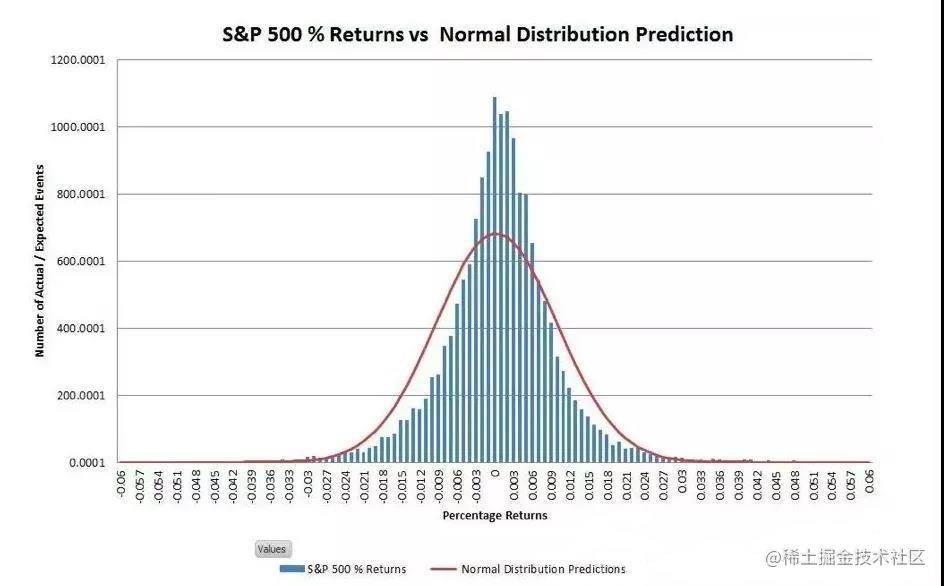2.公式推导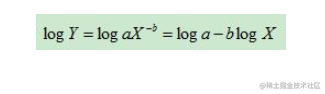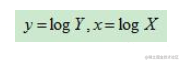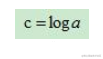c为常数，所以公式变成：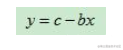3.可视化

4.代码实现

``````#!/usr/bin/env python
# -*-coding:utf-8 -*-

import matplotlib.pyplot as plt
import numpy as np
from sklearn import linear_model
from scipy.stats import norm

def DataGenerate():
X = np.arange(10, 1010, 10)  # 0-1，每隔着0.02一个数据  0处取对数,会时负无穷  生成100个数据点
noise=norm.rvs(0, size=100, scale=0.2)  # 生成50个正态分布  scale=0.1控制噪声强度
Y=[]
for i in range(len(X)):
Y.append(10.8*pow(X[i],-0.3)+noise[i])  # 得到Y=10.8*x^-0.3+noise

# plot raw data
Y=np.array(Y)
plt.title("Raw data")
plt.scatter(X, Y,  color='black')
plt.show()

X=np.log10(X)  # 对X，Y取双对数
Y=np.log10(Y)
return X,Y

def DataFitAndVisualization(X,Y):
# 模型数据准备
X_parameter=[]
Y_parameter=[]
for single_square_feet ,single_price_value in zip(X,Y):
X_parameter.append([float(single_square_feet)])
Y_parameter.append(float(single_price_value))

# 模型拟合
regr = linear_model.LinearRegression()
regr.fit(X_parameter, Y_parameter)
# 模型结果与得分
print('Coefficients: \n', regr.coef_,)
print("Intercept:\n",regr.intercept_)
# The mean square error
print("Residual sum of squares: %.8f"
% np.mean((regr.predict(X_parameter) - Y_parameter) ** 2))  # 残差平方和

# 可视化
plt.title("Log Data")
plt.scatter(X_parameter, Y_parameter,  color='black')
plt.plot(X_parameter, regr.predict(X_parameter), color='blue',linewidth=3)

# plt.xticks(())
# plt.yticks(())
plt.show()

if __name__=="__main__":
X,Y=DataGenerate()
DataFitAndVisualization(X,Y)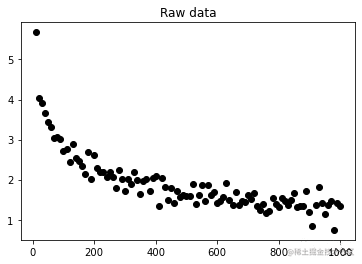Coefficients: [-0.32608517] Intercept: 1.0956477044386048 Residual sum of squares: 0.00303181